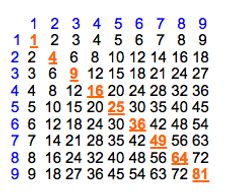## A NEW NOTATION FOR MULTIPLYING NUMBERS

#### The Generic FormatHere is the extended generic form of the Mighty Math system. In this example, we multiply the fictional number ABC times 123

#### One Quick ExampleHere we multiply 12 times 34 and get the answer 408 in short order. Open up the pamphlette below to see the benefits

#### The complete pamphlette belowThe method is detailed in two word documents below and one power point presentation for your edification.

#### Several More examplesHere is a sample of some examples using the Mighty Math Method.

#### The Most Important 36 NumbersThese are the most important numbers you'll ever learn, the times-table 36. Remember these, and you'll be better off than if you hadn't.

#### Another Layout of the 36